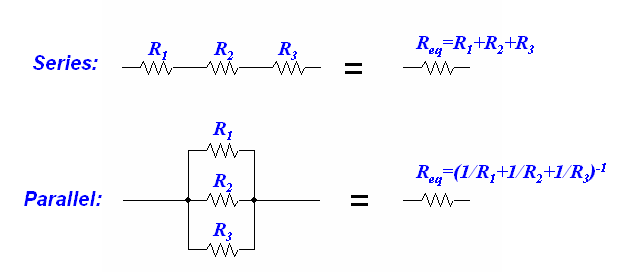# Question #886c6

May 28, 2014

When resistors are connected in parallel, they are each on their own path. This means that electricity can run through any one of them at a time without flowing through the others.

The total current of the circuit is the sum of the current flowing through each resistor. (${I}_{T} = {I}_{1} + {I}_{2} + \ldots$).

Each resistor slows only a portion of the current.

$\frac{1}{R} _ T = \frac{1}{R} _ 1 + \frac{1}{R} _ 2 + \ldots$

This is different from when resistors are connected in series, where there is only one path for electricity to take through each resistor in order.